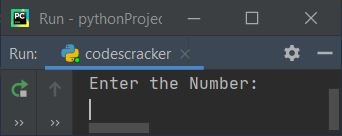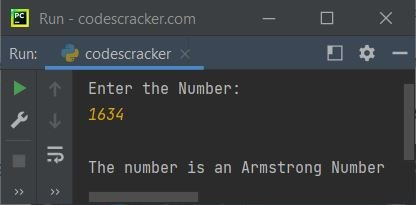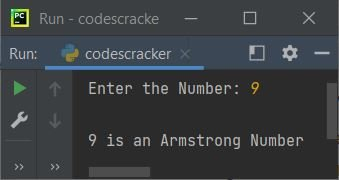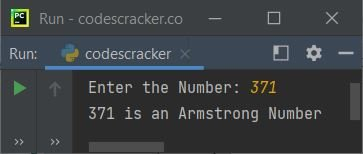# Python Program to Check Armstrong Number

In this article, we've created some programs in Python, to check whether a number entered by user is an Armstrong number or not. Here are the list of programs:

• Simple Program to Check Armstrong Number
• Check Armstrong Number using user-defined Function
• Using class

But before starting these programs, let me clear the thing about Armstrong number, that what it is.

### What is an Armstrong Number ?

A number that equals to the sum of its own digits, where each digit raised to the power of number of digits. For example, 153 is an Armstrong number, because:

```153 = 13 + 53 + 33
= 1 + 125 + 27
= 153```

The result (153) is equal to the number (153) itself. So it is an Armstrong number.

Note - Because the total number of digit in 153 is 3, so each of its digit raised to the power of 3.

Note - 1, 2, 3, 4, 5, 6, 7, 8, and 9 are all Armstrong numbers.

## Check Armstrong Number

To check whether a given number is an Armstrong number or not in Python, you have to ask from user to enter a number, then apply the formula, check and print the message as shown in the program given below:

```print("Enter the Number: ")
num = int(input())

temp = num
noOfDigit = 0
res = 0
while num>0:
num = int(num/10)
noOfDigit = noOfDigit+1
num = temp
while num>0:
rem = num%10
pow = 1
i = 0
while i<noOfDigit:
pow = pow*rem
i = i+1
res = res+pow
num = int(num/10)

if res==temp:
print("\nThe number is an Armstrong Number")
else:
print("\nThe number is not an Armstrong Number")```

Here is the initial output produced by this Python program:Now supply the input say 1634 and press `ENTER` key to check whether it is an armstrong number or not. Here is its sample run with this user input:Since 1634 is a four-digit number, therefore:

```1634 = 14 + 64 + 34 + 44
= 1 + 1296 + 81 + 256
= 1297 + 337
= 1634```

In above program, the following block of code:

```while num>0:
num = int(num/10)
noOfDigit = noOfDigit+1```

is used to count the total number of digits of given number. The dry run of this block of code with user input 1634 goes like:

• Initial value, noOfDigit=0
• The condition, num>0 or 1634>0 evaluates to be true, therefore program flow goes to the body of while loop and executes both the statements
• That is, int(num/10) or int(1634/10) or 163 initialized to num. So num=163 now. And noOfDigit+1 or 0+1 or 1 gets initialized to noOfDigit. So noOfDigit=1
• Now the condition of while loop again gets evaluated, that is the condition num>0 or 163>0 evaluates to be true again, therefore program flow goes inside its body and executes that two statements again. This process continues, until the condition evaluates to be false
• In this way, after exiting from this loop, the variable noOfDigit holds its value as 4

And the following block of code:

```while i<noOfDigit:
pow = pow*rem
i = i+1```

is used to calculate the value of digit's raised to the power of number of digit. The dry run of this block of code goes like:

• Initial values, i=0, noOfDigit=4, pow=1, rem=4 (because 1634%10 is equal to 4)
• Now the condition i<noOfDigit or 0<4 evaluates to be true, therefore program flow goes inside its body
• And pow*rem or 1*4 or 4 gets initialized to pow. So pow=4, and the value of i gets incremented by 1. So i=1
• Since the condition at second evaluation, that is i<noOfDigit or 1<4 evaluates to be true again, therefore program flow again goes inside the loop and pow*rem or 4*4 or 16 initialized to pow. So pow=16 and the value of i=2
• At third time also, the condition i<noOfDigit or 2<4 evaluates to be true, therefore program flow again goes inside the loop. This process continues until the condition evaluates to be true
• In this way, after exiting from this loop, the variable pow holds its value as 1*4*4*4*4 or 256 that is equal to 44, where 4 is the digit (last digit of number) and power 4 indicates to total number of digit

After second evaluation of this block of code, pow holds its value as 3*3*3*3, at third time pow holds 6*6*6*6 and at fourth time pow holds 1*1*1*1

#### Modified Version of Previous Program

This is the modified version of previous program. In this program, we've used end to skip printing of an automatic newline using print(). And rem**noOfDigit is used to find remnoOfDigit value. The str() method is used to convert any type of value to string type value. Rest of the things are similar to previous program.

```print("Enter the Number: ", end="")
num = int(input())

temp = num
noOfDigit = len(str(num))
res = 0
while num>0:
rem = num%10
res = res + (rem ** noOfDigit)
num = int(num/10)

if res==temp:
print("\n" +str(temp)+ " is an Armstrong Number")
else:
print("\n" +str(temp)+ " is not an Armstrong Number")```

Here is its sample run with user input, 9:## Check Armstrong Number using Function

This program uses used-defined function named checkArmstrongNum() to check whether a number entered by user at run-time is an Armstrong number or not.

```def checkArmstrongNum(x):
noOfDigit = 0
res = 0
temp = x
while x>0:
x = int(x/10)
noOfDigit = noOfDigit + 1
x = temp
while x>0:
rem = x%10
pow = 1
i = 0
while i<noOfDigit:
pow = pow * rem
i = i + 1
res = res + pow
x = int(x/10)

if res==temp:
return 1
else:
return 0

print("Enter the Number: ", end="")
num = int(input())

chk = checkArmstrongNum(num)
if chk==1:
print(num, "is an Armstrong Number")
else:
print(num, "is not an Armstrong Number")```

Here is its sample run with user input, 371:Here is another sample run with user input, 532:## Check Armstrong Number using Class

This is the last program of this article on checking Armstrong number, using class, an object-oriented feature of Python:

```class CodesCracker:
def checkArmstrongNum(self, x):
noOfDigit = len(str(x))
res = 0
temp = x
while x>0:
rem = x%10
res = res + (rem ** noOfDigit)
x = int(x/10)
if res==temp:
return 1
else:
return 0

print("Enter the Number: ", end="")
num = int(input())

obj = CodesCracker()
chk = obj.checkArmstrongNum(num)

if chk==1:
print(num, "is an Armstrong Number")
else:
print(num, "is not an Armstrong Number")```

An object obj is created of type CodesCracker class to access its member function named checkArmstrongNum() using dot (.) operator.

#### Same Program in Other Languages

Python Online Test

« Previous Program Next Program »